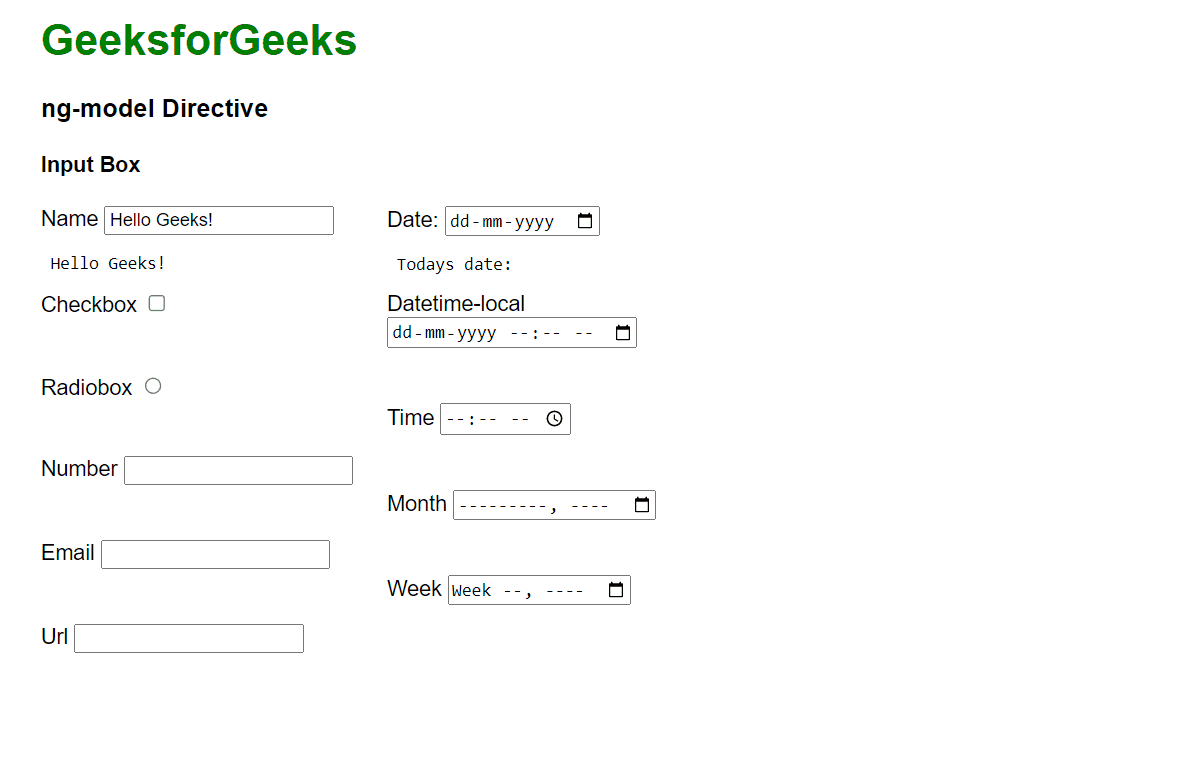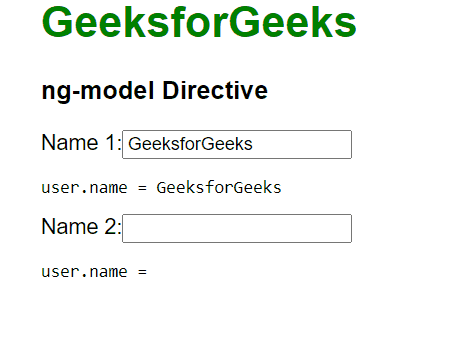GFG App
Open AppBrowser
Continue

# AngularJS ng-model Directive

The ngModel directive is a directive that is used to bind the values of the HTML controls (input, select, and textarea) or any custom form controls, and stores the required user value in a variable and we can use that variable whenever we require that value. It also is used during form validations. The various form input types (text, checkbox, radio, number, email, URL, date, datetime-local time, month, week) can be used with the ngModel directive. This directive is supported by <input>, <select> & <textarea>.

Syntax:

```<element ng-model="">
Content...
</element>```

Example 1: This example describes the basic usage of the ng-model Directive in AngularJS.

## HTML

 ` ` `<``html``> ` ` `  `<``head``> ` `    ``<``title``>AngularJS ng-model Directive ` `    ``<``script` `src``= ` `"https://ajax.googleapis.com/ajax/libs/angularjs/1.6.9/angular.min.js"``> ` `    `` ` `    ``<``style``> ` `        ``body { ` `            ``font-family: Arial; ` `        ``} ` `        ``h1 { ` `            ``color: green; ` `        ``} ` `        ``.column { ` `            ``float: left; ` `            ``text-align: left; ` `            ``width: 49%; ` `        ``} ` `        ``.row { ` `            ``content: ""; ` `            ``display: table; ` `        ``} ` `    `` ` ` ` ` `  `<``body` `ng-app``=``"myApp"` `ng-controller``=``"myController"``> ` `    ``<``h1``>GeeksforGeeks ` `    ``<``h3``>ng-model Directive ` `    ``<``h4``>Input Box ` `    ``<``div` `class``=``"row"``> ` `        ``<``div` `class``=``"column"``> ` `            ``Name ` `            ``<``input` `type``=``"text"` `ng-model``=``"name"``> ` `            ``<``pre``> {{ name }} Checkbox ` `            ``<``input` `type``=``"checkbox"` `ng-model``=``"check"``> ` `            ``<``pre``> {{ check }} Radiobox ` `            ``<``input` `type``=``"radio"` `ng-model``=``"choice"``> ` `            ``<``pre``> {{ choice }} Number ` `            ``<``input` `type``=``"number"` `ng-model``=``"num"``> ` `            ``<``pre``> {{ num }} Email ` `            ``<``input` `type``=``"email"` `ng-model``=``"mail"``> ` `            ``<``pre``> {{ mail }} Url ` `            ``<``input` `type``=``"url"` `ng-model``=``"url"``> ` `            ``<``pre``> {{ url }} ` `        `` ` `        ``<``div` `class``=``"column"``> ` `            ``Date: ` `            ``<``input` `type``=``"date"` `ng-model``=``"date1"`  `                   ``(change)="log(date1)"> ` `            ``<``pre``> Todays date:{{ date1 }} Datetime-local ` `            ``<``input` `type``=``"datetime-local"` `ng-model``=``"date2"``> ` `            ``<``pre``> {{ date2 }} Time ` `            ``<``input` `type``=``"time"` `ng-model``=``"time1"``> ` `            ``<``pre``> {{ time1}} Month ` `            ``<``input` `type``=``"month"` `ng-model``=``"mon"``> ` `            ``<``pre``> {{ mon }} Week ` `            ``<``input` `type``=``"week"` `ng-model``=``"we"``> ` `            ``<``pre``> {{ we }} ` `        `` ` `    `` ` ` ` `<``script``> ` `    ``var app = angular.module('myApp', []); ` `    ``app.controller('myController', function (\$scope) { ` `        ``\$scope.name = "Hello Geeks!"; ` `        ``\$scope.check = ""; ` `        ``\$scope.rad = ""; ` `        ``\$scope.num = ""; ` `        ``\$scope.mail = ""; ` `        ``\$scope.url = ""; ` `        ``\$scope.date1 = ""; ` `        ``\$scope.date2 = ""; ` `        ``\$scope.time1 = ""; ` `        ``\$scope.mon = ""; ` `        ``\$scope.we = ""; ` `        ``\$scope.choice = ""; ` `        ``\$scope.c = function () { ` `            ``\$scope.choice = true; ` `        ``}; ` `    ``}); ` ` ` ``

Output:In order to make URL and email print, we have to write a valid email/URL only then it would get printed. In the case of printing of date, and time using ngmodel, then we have to fill in all the fields in the input box. The radio button once selected won’t get deselected since, in the function of “c”, we are setting the value of choice as true.

HTML forms using the ng-model directive: We can define ngModel in the following way by writing the below code in the app.component.html file.

```<div class="form-group">
<label for="phone">mobile</label>
<form>
<input type="text"
id="phone"
ngModel name="phone"
#phone="ngModel"
placeholder="Mobile">
</form>
<pre>{{ phone.value }}</pre>
</div>```

The ngModel directive stores a variable by reference, not value. Usually in binding inputs to models that are objects (e.g. Date) or collections (e.g. arrays). The phone object created has many fields which are used for validation purposes. The following is the list of classes for validation purposes:

• ng-touched: It shows that field has been touched.
• ng-untouched: It shows that field has not been touched yet.
• ng-valid: It specifies that the field content is valid.
• ng-invalid: It specifies that the field content is not valid.
• ng-dirty:  It illustrates that the field has been modified.
• ng-pristine: It represents that the field has not been modified yet.

Binding ngModel with getter and setter: Whenever a function is called with zero arguments then it returns a representation of the model. And when called with a parameter it sets the value. Since ngModel refers to address that is why it does not save the changed value in the object itself rather it saves it in some internal state (variable-name.value). It will be useful if we keep a practice of using getter and setter for models when there is an internal representation as the getter and setter function gets more often called as compared to the rest of the parts of the code.

Syntax:

`ng-model-options="{ getterSetter: true }"`

We can add this to the input element.

Example 2: This example describes the ng-model Directive in AngularJS, where we have bound the ngModel directive with getter and setter.

## HTML

 ` ` `<``html``> ` ` `  `<``head``> ` `    ``<``title``>AngularJS ng-model Directive ` `    ``<``script` `src``= ` `"https://ajax.googleapis.com/ajax/libs/angularjs/1.6.9/angular.min.js"``> ` `    `` ` ` `  `    ``<``style``> ` `        ``body { ` ` `  `            ``font-family: Arial; ` `            ``margin-left: 45px; ` `        ``} ` ` `  `        ``h1 { ` `            ``color: green; ` `        ``} ` `    `` ` ` ` ` `  `<``body` `ng-app``=``"myApp"``> ` `    ``<``h1``>GeeksforGeeks ` `    ``<``h3``>ng-model Directive ` `    ``<``div` `ng-controller``=``"myController"``> ` `        ``<``form``> ` `            ``Name 1:<``input` `type``=``"text"` `name``=``"Name"`  `                ``ng-model``=``"user.name"`  `                ``ng-model-options``=``"{ getterSetter: true }"` `/> ` `            ``<``pre``>user.name =  ` `                ``<``span` `ng-bind``=``"user.name()"``> ` `            `` ` `            ``Name 2:<``input` `type``=``"text"` `name``=``"Name1"`  `                ``ng-model``=``"user.name1"`  `                ``ng-model-options``=``"{ getterSetter: true }"` `/> ` `            ``<``pre``>user.name = ` `                ``<``span` `ng-bind``=``"user.name1()"``> ` `            `` ` `        `` ` `    `` ` `    ``<``script``> ` `        ``angular.module('myApp', []) ` `            ``.controller('myController', ['\$scope', function (\$scope) { ` `                ``name = 'GeeksforGeeks'; ` `                ``name1 = ""; ` `                ``\$scope.user = { ` `                    ``name: function (Name) { ` `                        ``return arguments.length ? (name = Name) : name; ` `                    ``}, ` `                    ``name1: function (Name1) { ` `                        ``return arguments.length ? (name1 = Name1) : name1; ` `                    ``} ` `                ``}; ` `            ``}]); ` `    `` ` ` ` ` `  ``

Output: Here, we have initialized name 1 by the string GeeksforGeeks and name 2 by an empty string.My Personal Notes arrow_drop_up